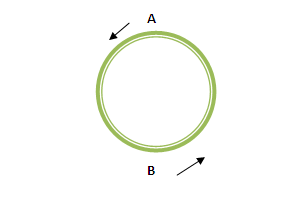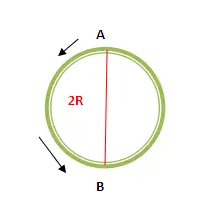# What is the difference between distance and displacement?

Distance is the length actually traveled on a route while Displacement is the shortest distance (straight line distance) between the initial point and final point of the traveled path.

Distance is a scalar quantity and required to calculate speed. On the other hand, displacement is a vector quantity that has both magnitude and direction. Displacement is used to calculate the velocity of an object in motion.

## Understanding the difference between distance and displacement with an example

Now we will explain the difference between distance and displacement with an example.figure 1 [ Run from A to B and B to A ]

So if anybody runs a completely circular route once then the Distance traveled is equal to the circumference of the circle i.e. 2.π. R where R is the radius of the circle (and π is 22/7).

Say, for the circular track in figure 1 above, when someone runs from A to B and then B to A (following the given circular track) distance traveled can be obtained by the above formula, distance = 2.π. R

At the same time the Displacement = 0 because the final point(A) is the same as the initial point(A).figure 2 [ Run from A to B ]

Similarly, if the runner runs half of the circular track (A to B only) then the Distance traveled is half of the circumference of the circle = π.R

And the Displacement, in this case, is AB that is equal to 2R or the Diameter of the circle.

Related Posts you must read

What is the difference between distance and displacement?
Scroll to top
error: physicsTeacher.in